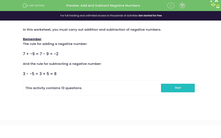# Add and Subtract Negative Numbers

In this worksheet, students carry out addition and subtraction of negative numbers.Key stage:  KS 3

Curriculum topic:   Number

Curriculum subtopic:   Use Four Operations for All Numbers

Difficulty level:#### Worksheet Overview

In this worksheet, you must carry out addition and subtraction of negative numbers.

Remember

The rule for adding a negative number:

7 + -9 = 7 - 9 = -2

And the rule for subtracting a negative number:

3 - -5 = 3 + 5 = 8

### What is EdPlace?

We're your National Curriculum aligned online education content provider helping each child succeed in English, maths and science from year 1 to GCSE. With an EdPlace account you’ll be able to track and measure progress, helping each child achieve their best. We build confidence and attainment by personalising each child’s learning at a level that suits them.

Get started